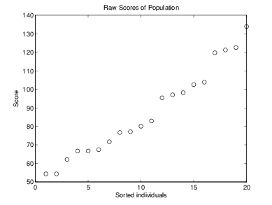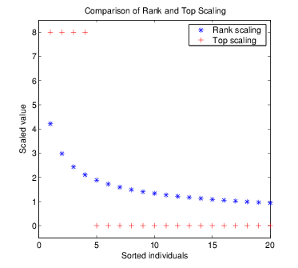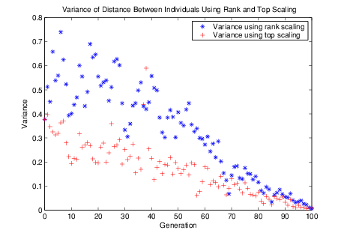## Fitness Scaling

### Scaling the Fitness Scores

Fitness scaling converts the raw fitness scores that are returned by the fitness function to values in a range that is suitable for the selection function. The selection function uses the scaled fitness values to select the parents of the next generation. The selection function assigns a higher probability of selection to individuals with higher scaled values.

The range of the scaled values affects the performance of the genetic algorithm. If the scaled values vary too widely, the individuals with the highest scaled values reproduce too rapidly, taking over the population gene pool too quickly, and preventing the genetic algorithm from searching other areas of the solution space. On the other hand, if the scaled values vary only a little, all individuals have approximately the same chance of reproduction and the search will progress very slowly.

The default fitness scaling option, `Rank`, scales the raw scores based on the rank of each individual instead of its score. The rank of an individual is its position in the sorted scores: the rank of the most fit individual is 1, the next most fit is 2, and so on. The rank scaling function assigns scaled values so that

• The scaled value of an individual with rank n is proportional to $1/\sqrt{n}$.

• The sum of the scaled values over the entire population equals the number of parents needed to create the next generation.

Rank fitness scaling removes the effect of the spread of the raw scores.

The following plot shows the raw scores of a typical population of 20 individuals, sorted in increasing order.The following plot shows the scaled values of the raw scores using rank scaling.Because the algorithm minimizes the fitness function, lower raw scores have higher scaled values. Also, because rank scaling assigns values that depend only on an individual's rank, the scaled values shown would be the same for any population of size 20 and number of parents equal to 32.

### Comparing Rank and Top Scaling

To see the effect of scaling, you can compare the results of the genetic algorithm using rank scaling with one of the other scaling options, such as `Top`. By default, top scaling assigns 40 percent of the fittest individuals to the same scaled value and assigns the rest of the individuals to value 0. Using the default selection function, only 40 percent of the fittest individuals can be selected as parents.

The following figure compares the scaled values of a population of size 20 with number of parents equal to 32 using rank and top scaling.Because top scaling restricts parents to the fittest individuals, it creates less diverse populations than rank scaling. The following plot compares the variances of distances between individuals at each generation using rank and top scaling.Watch now